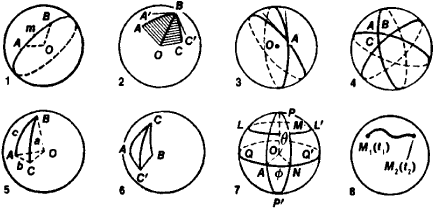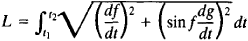# Spherical Geometry

Also found in: Dictionary, Thesaurus, Wikipedia.
Related to Spherical Geometry: spherical trigonometry, Spherical coordinates

## spherical geometry

[′sfir·ə·kəl je′äm·ə·trē]
(mathematics)
The geometry of points on a sphere.
McGraw-Hill Dictionary of Scientific & Technical Terms, 6E, Copyright © 2003 by The McGraw-Hill Companies, Inc.
The following article is from The Great Soviet Encyclopedia (1979). It might be outdated or ideologically biased.

## Spherical Geometry

the mathematical discipline that studies geometric figures on a sphere, just as plane geometry studies geometric figures in the plane.

Every plane section of a sphere is a circle. If the plane passes through the center O of the sphere, the resulting section is called a great circle. Only one great circle can be drawn through two points A and B on a sphere (Figure 1,1), unless the points are antipodal. The great circles of a sphere are geodesies, and thus play in spherical geometry a role analogous to that of straight lines in plane geometry. Whereas, however, any line segment is the shortest distance between its end points, only the shorter, or minor, arc of a great circle determined by two nonantipodal points has this property. Spherical geometry differs from plane geometry in many other respects as well. For example, there are no parallel geodesies in spherical geometry, since two great circles always intersect; in fact, they always intersect at two points.

The length of the segment AB on a sphere—that is, the length of the arc AmB (Figure 1,1) of a great circle—can be measured by means of the angle AOB, which is proportional to the length of the arc. The measure of the angle ABC (Figure 1,2) formed on a sphere by the intersection of two great circles is the angle A’BC’ between the tangents to the corresponding arcs at the point of intersection B and is thus equal to the measure of the dihedral angle formed by the planes OBA and OBC.

Four lunes are formed by the intersection of two great circles on a sphere (Figure 1,3). A lune is determined by specifying its angle. The area of a lune is given by the formula S = 2R2A, where R is the radius of the sphere and A is the angle of the lune in radians.

Three great circles that do not intersect in a single pair of antipodal points form eight spherical triangles on the sphere (Figure 1,4). If the elements, that is, the angles and sides, of one of the triangles are known, the elements of the other triangles can be easily found. For this reason, the relations between the elements of only one triangle are usually considered; the sides of this triangle are minor arcs of the great circles. The measures of the sides a, b, and c of a spherical triangle are equal to the measures of the corresponding plane angles of the trihedral angle OABC (Figure 1,5); the measures of the angles A, B, and C of the triangle are equal to the measures of the corresponding dihedral angles of the trihedral angle.

Spherical triangles differ in several respects from triangles in the plane. For example, in the case of spherical triangles, the three necessary and sufficient conditions for the congruence of plane triangles are supplemented by a fourth necessary and sufficient condition: two triangles are congruent if their corresponding angles are equal (there are no similar triangles on a sphere).Figure 1

Congruent spherical triangles are spherical triangles that can be made to coincide by means of motions on the sphere. It therefore follows that congruent spherical triangles have equal elements and the same orientation. Triangles with equal elements and different orientation are said to be symmetric—for example, the triangles AC’C and BCC’ in Figure 1,6.

In any spherical triangle formed by minor arcs of great circles, the sum of any two sides is greater than the third side, and the difference between any two sides is less than the third side. The sum of the sides is always less than 2π. The sum of the angles of a spherical triangle is always less than 3π and greater than π. The difference s – π = ∊, where s is the sum of the angles of a spherical triangle, is called the spherical excess. The area of a spherical triangle is given by the formula S = R2∊, where R is the radius of the sphere.

The position of any point on the sphere is completely determined by specifying two numbers. A description follows of a way by which these numbers, or coordinates, may be defined (Figure 1,7).

Some great circle QQ’ is taken as the equator. One of the two points, say P, where the diameter PP’ of the sphere perpendicular to the plane of the equator intersects the surface of the sphere, is taken as the pole. Finally, a great semicircle PAP’ emanating from the pole is taken as the zero meridian. The great semicircles of a sphere emanating from P are called meridians, and the small circles parallel to the equator are called parallels. The angle θ = POM, called the polar distance, is taken as one of the coordinates of a point M on the sphere, and the angular distance ɸ = AON between the zero meridian and the meridian through M is taken as the other coordinate, which is called the longitude and is reckoned counterclockwise.

The use of coordinates on the sphere permits spherical figures to be studied by means of the methods of analytic geometry. For example, the two equations

θ= f(t) ɸ = g(t)

or the single equation

F(θ, ɸ) = 0

involving the coordinates θ and ɸ define a curve on the sphere. The length L of an arc M1M2 of the curve is calculated by means of the formulawhere t1 and t2 are values of the parameter t at the end points M1 and M2 of M1M2 (Figure 1,8).

### REFERENCES

Stepanov, N. N. Sfericheskaia trigonometriia, 2nd ed. Leningrad-Moscow, 1948.
Entsiklopediia elementarnoi matematiki, book 4: Geometriia. Moscow, 1963.
References in periodicals archive ?
Scherzer, "Exact reconstruction in photoacoustic tomography with circular integrating detectors II: spherical geometry," Mathematical Methods in the Applied Sciences, vol.
There are plenty of curves in spherical geometry. The 'lines of latitude' are circles with their centre on the earth's axis of rotation.
The spherical geometry due to its one-dimensional simplicity was approached through a finite difference (FD) method solved implicitly by a Crank-Nicolson algorithm for variable coefficients .
The logic goes more or less like this: In a closed three-dimensional space, if all loops of thread can be pulled tight to a point, mathematicians know that the only one of the eight geometries that can fit the space is spherical geometry. That means that no matter how convoluted the space appears, it must simply be a distorted version of the hypersphere.
However, as McCluskey points out, these anthologies lacked the mathematical principles of spherical geometry (unlike Ptolemy's works); their tables of the heavenly bodies described only mean (average) motions and not variations therefrom; and they placed stars inaccurately within the constellations.
Now it turns out that there are nonspherieal figures that can represent visible But that is all right, because using those representatives will not lead us to assign to visibles any properties incompatible with those of spherical geometry.
She recognized this definition to be incorrect for triangles in Spherical Geometry. By her own admission, she "wanted the definition to stand in both geometries," so she changed her definition of triangle to "a polygon with exactly 3 sides." As a class, they had previously included "straight" lines and "closed" figure in their definition of polygon.
They focus on a special class of wavelets and their applications, and develop special wavelet bases that are related to special functions in one part and adapted to spherical geometry in another.
In , the covariant metric tensor exterior to a homogeneous time varying distribution of mass within regions of spherical geometry is defined as:
Modeling large-scale atmosphere-ocean processes implies the use of the spherical geometry for the formulation of the governing equations.
Among specific topics are an immersed boundary method for simulating compressible viscous flow in complex geometries, the numerical study of battle damaged two-dimensional wings, the meridional flow of grounded abyssal currents on a sloping bottom in spherical geometry, acoustic frequency for bubble size correlation using acoustic emissions, and using heart blood flow analysis in clinical practice.
The contours .sub.[phi][C.sub.[pi]/2] and .sub.[phi][C.sub.[theta]] are regarded as a natural extension of the above geometric figures to spherical geometry, however, they are not so simple (see, Figure 2.2).

Site: Follow: Share:
Open / Close